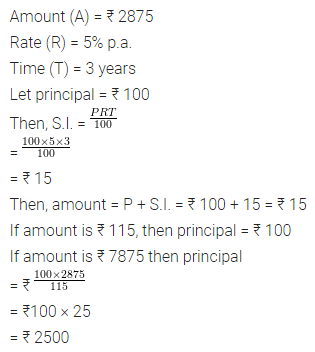# ML Aggarwal Class 7 ICSE Maths Model Question Paper 2

## ML Aggarwal Class 7 ICSE Maths Model Question Paper 2

(Based on Chapters 4 to 7)
Time allowed: 1 Hour
Maximum Marks: 25

Instructions

• Questions 1-2 carry 1 mark each
• Questions 3-5 carry 2 marks each
• Questions 6-8 carry 3 marks each
• Questions 9-10 carry 4 marks each.

Choose the correct answer from the given four options (1-2):
Question 1.
The expression $$\left( \frac { 1 }{ 3 } \right) ^{ 9 }$$ × (34)2 is equal to
(a) 39
(b) 3
(c) $$\left( \frac { 1 }{ 3 } \right) ^{ 2 }$$
(d) $$\frac { 1 }{ 3 }$$
Solution:Question 2.
On selling an article for ₹ 100, Renu gains ₹ 20. Her gain percentage is
(a) 25%
(b) 20%
(c) 16$$\frac { 2 }{ 3 }$$ %
(d) 15%
Solution: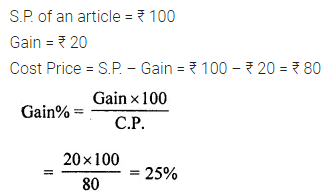Question 3.
Ravi donated ₹ 36 to a blind school and Rashmi donated ₹ 63 to an orphanage. Who donated more money?
Solution:Question 4.
Find the cardinal number of the set A where A = {x | x is a two-digit number, the sum of whose digits is 11}.
Solution:Question 5.
Find the fourth proportional to 8, 24, 25.
Solution:Question 6.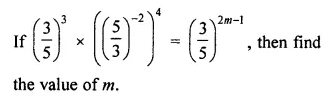Solution: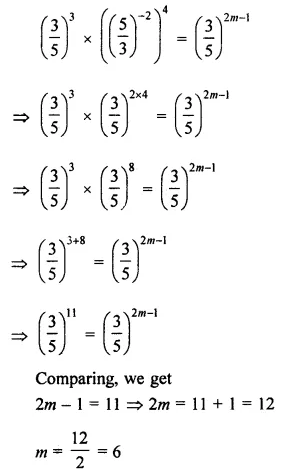Question 7.
18% of the apples in a basket go bad. If there are 123 good apples in the basket, find the total number of apples in the basket.
Solution:Question 8.
A natural number has been divided into two parts in the ratio 8 : 5. If the difference of two parts is 24, find the number and the two parts.
Solution:Question 9.
If 2.5 kg of apples cost ₹ 187.5, then how much quantity of apples can be bought for ₹ 330?
Solution: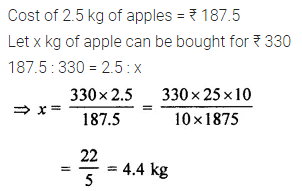Question 10.
What sum of money will amount to ₹ 2875 in 3 years at 5% per annum simple interest?
Solution: